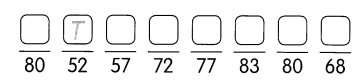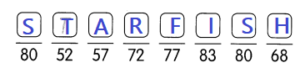Question 1.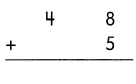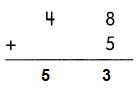Explanation:
Regroup the numbers in ones place at first,
8 + 5 = 13
then add the number in tens.
40 + 13 = 53
So, 48 + 5 = 53

Question 2.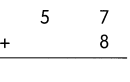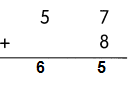Explanation:
Regroup the numbers in ones place at first,
7 + 8 = 15
then add the number in tens.
15 + 50 = 65
So, 57 + 8 = 65

Question 3.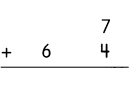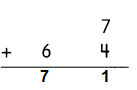Explanation:
Regroup the numbers in ones place at first,
7 +4 = 11
then add the number in tens.
60 + 11
So, 64 + 7 = 71

Question 4.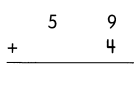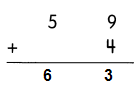Explanation:
Regroup the numbers in ones place at first,
9 + 4 = 13
then add the number in tens.
50 + 13 = 63
So, 59 + 4 = 63

Question 5.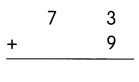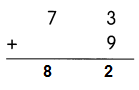Explanation:
Regroup the numbers in ones place at first,
3 + 9 = 12
then add the number in tens.
70 + 12 = 82
So, 73 + 9 = 82

Question 6.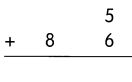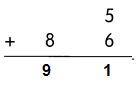Explanation:
Regroup the numbers in ones place at first,
5 + 6 = 11
then add the number to tens.
80 + 11 = 91
So, 86 + 5 = 91

Fill in the missing numbers.

Question 7.
4 + 66 = ____________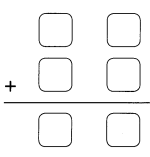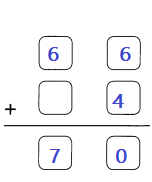Explanation:
Regroup the numbers in ones place at first,
4 + 6 = 10
then add the number to tens.
60 + 10 = 70
So, 66 + 4 = 70

Question 8.
89 + 8 = _____________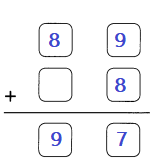Explanation:
Regroup the numbers in ones place at first,
9 + 8 = 17
then add the number in tens.
80 + 17 = 97
So, 89 + 8 = 97

Question 9.
Matt drops a ball into the number machine below. What happens to the number on the ball? Write the number in the ○.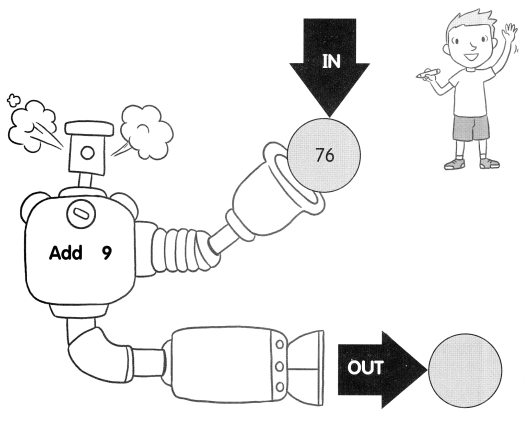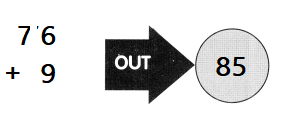Explanation:
Matt drops a ball consists of number 76 into the machine,
the number in the ball adds with 9 in the machine.
The out put of the ball is 85.
76 + 9 = 85

Question 10.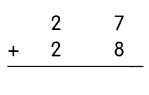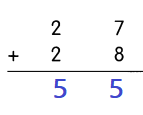Explanation:
Regroup the numbers in ones place at first,
7 + 8 = 15
then regroup the number in tens.
20 + 20 = 40
Add the sums of ones and tens
So, 40 + 15 = 55

Question 11.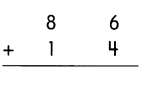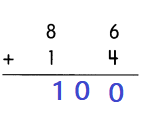Explanation:
Regroup the numbers in ones place at first,
6 + 4 = 10
then regroup the number in tens.
80 + 10 = 90
Add the sums of ones and tens
So, 90 + 10 = 100

Question 12.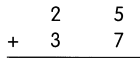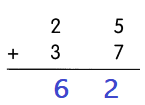Explanation:
Regroup the numbers in ones place at first,
5 + 7 = 12
then regroup the number in tens.
20 + 30 = 50
Add the sums of ones and tens
So, 50 + 12 = 62

Question 13.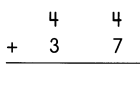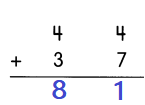Explanation:
Regroup the numbers in ones place at first,
4 + 7 = 11
then regroup the number in tens.
40 + 30 = 70
Add the sums of ones and tens
So, 70 + 11 = 81

Question 14.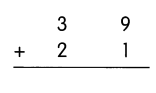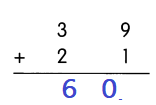Explanation:
Regroup the numbers in ones place at first,
9 + 1 = 10
then regroup the number in tens.
30 + 20 = 50
Add the sums of ones and tens
So, 50 + 10 = 60

Question 15.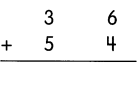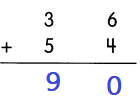Explanation:
Regroup the numbers in ones place at first,
6 + 4 = 10
then regroup the number in tens.
30 + 50 = 80
Add the sums of ones and tens
So, 80 + 10 = 90

Fill in the missing numbers.

Question 16.
19 + 14 = ____________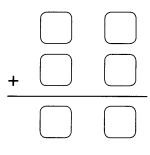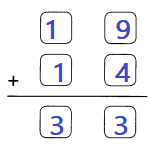Explanation:
Regroup the numbers in ones place at first,
9 + 4 = 13
then regroup the number in tens.
10 + 10 = 20
Add the sums of ones and tens
So, 20 + 13 = 33

Question 17.
58 + 36 = ___________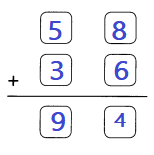Explanation:
Regroup the numbers in ones place at first,
8 + 6 = 14
then regroup the number in tens.
50 + 30 = 80
Add the sums of ones and tens
So, 80 + 14 = 94

Question 18.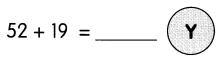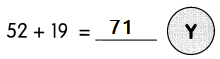Explanation:
Regroup the numbers in ones place at first,
2 + 9 = 11
then regroup the number in tens.
50 + 10 = 60
Add the sums of ones and tens
So, 60 + 11 = 71

Question 19.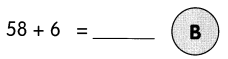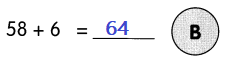Explanation:
Regroup the numbers in ones place at first,
8 + 6 = 14
then add the number in tens.
So, 50 + 14 = 64

Question 20.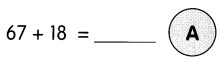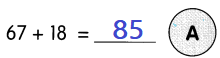Explanation:
Regroup the numbers in ones place at first,
8 + 7 = 15
then regroup the number in tens.
60 + 10 = 70
Add the sums of ones and tens
So, 70 + 15 = 85

Question 21.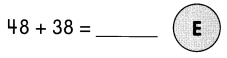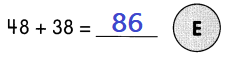Explanation:
Regroup the numbers in ones place at first,
8 + 8 = 16
then regroup the number in tens.
40 + 30 = 70
Add the sums of ones and tens
So, 70 + 16 = 86

Question 22.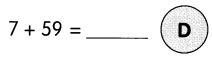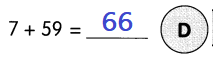Explanation:
Regroup the numbers in ones place at first,
7 + 9 = 16
then add the number in tens.
50 + 16 = 66

Question 23.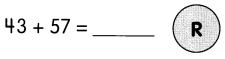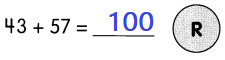Explanation:
Regroup the numbers in ones place at first,
3 + 7 = 10
then regroup the number in tens.
50 + 40 = 90
Add the sums of ones and tens
So, 90 + 10 = 100

Question 24.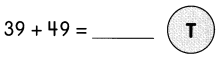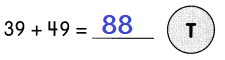Explanation:
Regroup the numbers in ones place at first,
9 + 9 = 18
then regroup the number in tens.
30 + 40 = 70
Add the sums of ones and tens
So, 70 + 18 = 88

Question 25.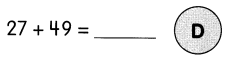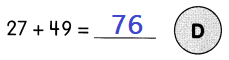Explanation:
Regroup the numbers in ones place at first,
7 + 9 = 16
then regroup the number in tens.
20 + 40 = 60
Add the sums of ones and tens
So, 60 + 16 = 76

Question 26.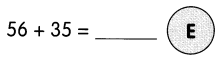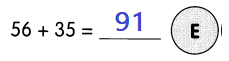Explanation:
Regroup the numbers in ones place at first,
5 + 6 = 11
then regroup the number in tens.
50 + 30 = 80
Add the sums of ones and tens
So, 80 + 11 = 91

What toy is named after President Theodore Roosevelt?

Match the letters to the answers below to find out.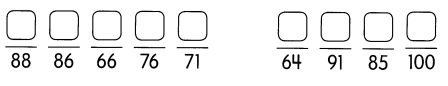TEDDY BEAR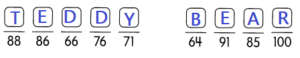Explanation:
From the above questions from Q18 to Q26,
arrange all the sums in an order to decode the the name of the president.
TEDDY BEAR is the toy named after President Theodore Roosevelt.

Question 27.
Ron drops a ball into a number machine. Which ball is it? Write the number in the ○.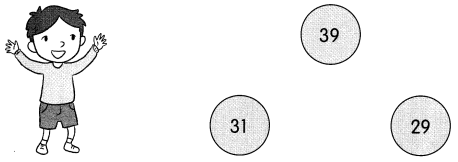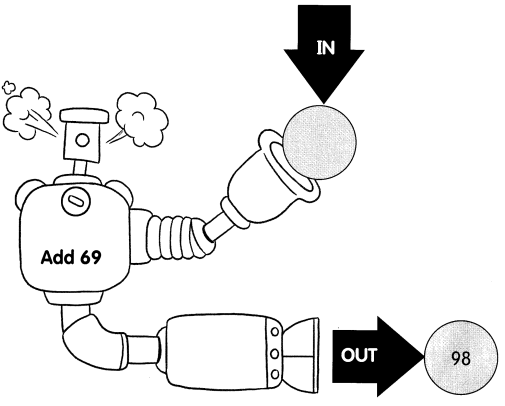29 + 69 = 98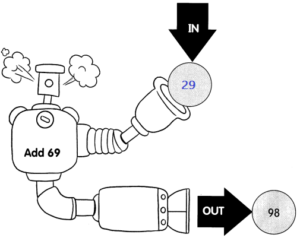Explanation:
Ron drops a ball number (x) into the machine,
the number(x) in the ball adds with 69 in the machine.
The out put of the ball is 98.
98 – 69 = 29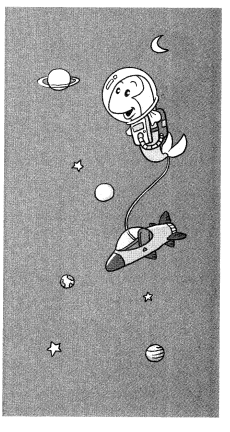Question 28.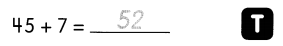Question 29.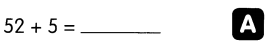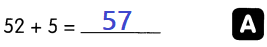Explanation:
Regroup the numbers in ones place at first,
2 + 5 = 7
then add the number in tens.
So, 50 + 7 = 57

Question 30.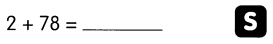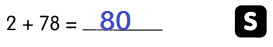Explanation:
Regroup the numbers in ones place at first,
2 + 8 = 10
then add the number in tens.
So, 70 + 10 = 80

Question 31.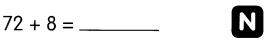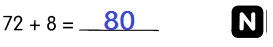Explanation:
Regroup the numbers in ones place at first,
2 + 8 = 10
then add the number in tens.
So, 70 + 10 = 80

Question 32.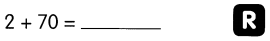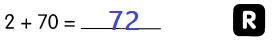Explanation:
Regroup the numbers in ones place at first,
2 + 0 = 2
then add the number in tens.
So, 70 + 2 = 72

Question 33.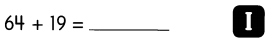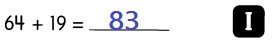Explanation:
Regroup the numbers in ones place at first,
4 + 9 = 13
then add the number in tens.
60 + 10 = 70
Add the sums of ones and tens place
70 + 13 = 83

Question 34.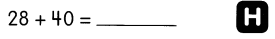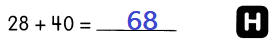Explanation:
Regroup the numbers in ones place at first,
8 + 0 = 8
then add the number in tens.
20 + 40 = 60
Add the sums of ones and tens place
60 + 8 = 68

Question 35.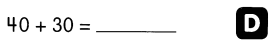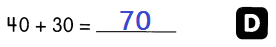Explanation:
Regroup the numbers in tens place,
as zeros are in ones place
40 + 30 = 70

Question 36.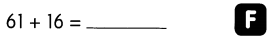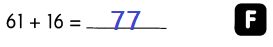Explanation:
Regroup the numbers in ones place at first,
1 + 6 = 7
then add the number in tens.
60 + 10 = 70
Add the sums of ones and tens place
70 + 7 = 77

Question 37.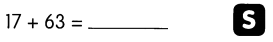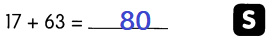Explanation:
Regroup the numbers in ones place at first,
7 + 3 = 10
then add the number in tens.
60 + 10 = 70
Add the sums of ones and tens place
70 + 10 = 80

Which fish do you find in space?

Match the letters to the answers below to find out.